Methods and formulas for 2 Variances

Select the method or formula of your choice.

Sample statistics

Minitab calculates the mean, standard deviation, and variance of both samples.

Formula

The standard deviation equals the square root of the variance.

Notation

TermDescription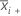mean of sample i
S2i variance of sample i
Xij jth measurement of the ith sample
ni size of sample i

Test for Bonett's method with balanced designs

Formula for the test statistic

When n1 = n2 , the test statistic is Z2. If the null hypothesis, ρ = ρ0 is true, then Z2 is distributed as a chi-square distribution with 1 degree of freedom. Z2 is given by:

where se(ρ0) is the standard error of the pooled kurtosis, which is given by:

where ri = ( ni - 3) / ni and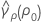is the pooled kurtosis, which is given by:

se20) can also be expressed in terms of the kurtosis values of the individual samples,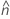, as follows:

where:

Formula for p-value

Let z2 be the value of Z2 that is obtained from the data. Under the null hypothesis, H0: ρ = ρ0 , Z is distributed as the standard normal distribution. Therefore, the p-values for the alternative hypotheses (H1) are given by the following.

Hypothesis P-value
H1: ρ0 ≠ ρ0 P = 2P(Z > |z|)
H1: ρ0 > ρ0 P = P(Z > z)
H1: ρ0 < ρ0 P = P(Z < z)

Notation

TermDescription
Sithe standard deviation of sample i
ρthe ratio of the population standard deviations
ρ0the hypothesized ratio of the population standard deviations
αthe significance level for the test = 1 - (the confidence level / 100)
nithe number of observations in sample i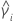the kurtosis value for sample i
Xijthe jth observation in sample i
mithe trimmed mean for sample i with trim proportions of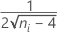Test for Bonett's method with unbalanced designs

Formula

When n1n2 , there is no test statistic. Rather, the p-value is calculated by inverting the confidence interval procedure. The p-value for the test is given by:

P = 2 min (αL, αU)

where αL is the smallest value of α for which the following holds:
and αU is the smallest value of α for which the following holds:

where cα is an equalizer constant described below and se(ρ0) is the standard error of the pooled kurtosis, which is given by:

where ri = (ni - 3) / ni andis the pooled kurtosis which is given by:

se(ρ0) can also be expressed in terms of the kurtosis values of the individual samples. For more information go to Test for Bonett's method with balanced designs.

Equalizer constant

The constant cα is included as a small-sample adjustment to mitigate the effect of unequal tail error probabilities in unbalanced designs. The value of cα is given by:

The constant vanishes when the designs are balanced, and its effect becomes negligible with increasing sample sizes.

Finding αL and αU

Finding αL and αU is equivalent to finding the roots of the functions L(z , n1 , n2 , S1 , S2 ) and L(z , n2 , n1 , S2 , S1 ), where L(z , n1 , n2 , S1 , S2) is given by:

Let:
For n1 < n2, do the following:
• Calculate zm and evaluate L(z, n1, n2, S1, S2).
• If L(zm)0, then find the root zL, of L(z, n1, n2, S1, S2) in the intervaland calculate αL = P( Z > zL).
• If L(zm) > 0, then the function L(z , n1, n2, S1, S2) has no root, and αL = 0.
For n1 > n2, do the following:
• Calculate L(0, n1, n2, S1, S2) = ln (S12 / S22).
• If L(0, n1, n2, S1, S2)0, then find the root z0, of L(z, n1, n2, S1, S2) in the interval [0, n2).
• If L(0, n1, n2, S1, S2) < 0, then find the root zL in the interval.
• Calculate αL = P( Z > zL).

To calculate αU, apply the previous steps using the function, L(z, n2, n1, S2, S1), instead of the function, L(z, n1, n2, S1, S2).

Notation

TermDescription
Sithe standard deviation of sample i
ρthe ratio of the population standard deviations
ρ0the hypothesized ratio of the population standard deviations
αthe significance level for the test = 1 - (the confidence level / 100)
zαthe upper α percentile point of the standard normal distribution
nithe number of observations in sample i
Xijthe jth observation in sample i
mithe trimmed mean for sample i with trim proportions ofConfidence interval for Bonett's method

Formula

Confidence intervals are obtained by inverting the test procedure. More specifically, Minitab solves the following equation for ρ:

where cα/2 is an equalizer constant (described below) and se(ρ) is the standard error of the pooled kurtosis (described below). Typically, this equation has two solutions, a solution, L < S1 / S2, and a solution U > S1 / S2. L is the lower confidence limit, and U is the upper confidence limit. For more information, go to Bonett's Method, which is a white paper that has simulations and other information about Bonett's Method.

The confidence limits for the ratio of the variances are obtained by squaring the confidence limits for the ratio of the standard deviations.

Equalizer constant

The constant cα is included as a small-sample adjustment to mitigate the effect of unequal tail error probabilities in unbalanced designs. The value of cα is given by:

The constant vanishes when the designs are balanced, and its effect becomes negligible with increasing sample sizes.

Standard error of the pooled kurtosis

se(ρ) is the standard error of the pooled kurtosis which is given by:

where ri = (ni - 3) / ni andis the pooled kurtosis which is given by:

se(ρ) can also be expressed in terms of the kurtosis values of the individual samples. For more information, see the section on the Test for Bonett's method with balanced designs.

Notation

TermDescription
αthe significance level for the test = 1 - (the confidence level / 100)
Sithe standard deviation of sample i
ρthe ratio of the population standard deviations
zα/2the upper α/2 percentile point of the standard normal distribution
nithe number of observations in sample i
Xijthe jth observation in sample i
mithe trimmed mean for sample i with trim proportions ofTest for Levene's method

Formula

Levene’s test is appropriate for continuous data. Levene’s test is not available for summarized data.

To test the null hypothesis that σ1 / σ2 = ρ with Levene’s test, Minitab performs a one-way ANOVA on the values Z1j and ρZ2j (where j = 1, …, n1 or n2).

The Levene's test statistic equals the value of the F-statistic in the resulting ANOVA table. The Levene's test p-value equals the p-value in this ANOVA table.

• H. Levene (1960). Contributions to Probability and Statistics. Stanford University Press, CA.
• M.B. Brown and A.B. Forsythe (1974). "Robust Tests for the Equality of Variance," Journal of the American Statistical Association, 69, 364–367.

Degrees of freedom

Under the null hypothesis, the test statistic follows an F-distribution with degrees of freedom DF1 and DF2.

DF1 = 1

DF2 = n1 + n2 – 2

Notation

TermDescription
Zij|Xi j η i|
TermDescription
j1, 2, …, ni
i1, 2
Xijindividual observations
ηimedian of sample i
σ1standard deviation of the first population
σ2standard deviation of the second population
n1size of the first sample
n2size of the second sample

Confidence intervals for Levene's method

Formula

For continuous data, Minitab calculates the confidence limits for the ratio (ρ) between the population standard deviations with the following formulas. To obtain limits for the ratio between population variances, square the values below.

When you specify an alternative hypothesis of Ratio ≠ hypothesized ratio, a 100(1–α)% confidence interval for ρ is given by:
• If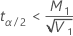, lower limit =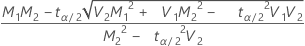If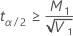, a lower limit does not exist

• If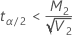, upper limit =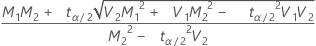If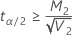, an upper limit does not exist

When you specify an alternative hypothesis of Ratio < hypothesized ratio, a 100(1 – α)% upper confidence bound for ρ is given by:
• If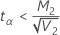, then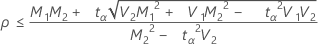• If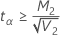, an upper bound does not exist
When you specify an alternative hypothesis Ratio > hypothesized ratio, a 100(1 – α)% upper confidence bound for ρ is given by:
• If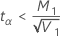, then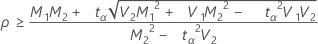• If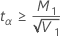, a lower bound does not exist

Notation

TermDescription
ηithe median of sample i
Zij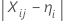where j = 1, 2, ... , ni and i = 1, 2, and Xij are individual observations
Mithe mean of Zij
Si2the sample variance of Zij
vi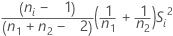ρσ1 / σ2
n1the size of the first sample
n2the size of the second sample

Test for the F-test method

The F-test is appropriate for normal data. To test the null hypothesis that σ1 / σ2 = ρ with the F-test, Minitab uses the following formulas.

Formula for degrees of freedom

Under the null hypothesis, the F-statistic follows an F-distribution with degrees of freedom DF1 and DF2.

DF1 = n1 – 1

DF2 = n2 – 1

Formula for p-value

The calculation of the p-value depends on the alternative hypothesis as follows.
• For a one-sided test with an alternative hypothesis of less than, the p-value equals the probability of obtaining an F-statistic that is equal to or less than the observed value from an F-distribution with degrees of freedom DF1 and DF2.
• For a two-sided test where the ratio is less than 1, the p-value equals two times the area under the F-curve less than the observed value from an F-distribution with degrees of freedom DF1 and DF2.
• For a two-sided test where the ratio is greater than 1, the p-value equals two times the area under the F-curve greater than the observed value from an F-distribution with degrees of freedom DF1 and DF2.
• For a one-sided test with an alternative hypothesis of greater than, the p-value equals the probability of obtaining an F-statistic that is equal to or greater than the observed value from an F-distribution with degrees of freedom DF1 and DF2.

Notation

TermDescription
ρσ1 / σ2
σ1standard deviation of the first population
σ2standard deviation of the second population
S21 variance of the first sample
S22 variance of the second sample
n1size of the first sample
n2size of the second sample

Confidence intervals for the F-test method

When the data follow a normal distribution, Minitab calculates the confidence bounds for the ratio (ρ) between the population standard deviations with the following formulas. To obtain bounds for the ratio between population variances, square the values below.

Formula

When you specify a "not equal" alternative hypothesis, a 100(1 – α)% confidence interval for ρ is given by:

When you specify a "less than" alternative hypothesis, a 100(1 – α)% upper confidence bound for ρ is given by:

When you specify a "greater than" alternative hypothesis, a 100(1 – α)% lower confidence bound for ρ is given by:

Notation

TermDescription
S1standard deviation of the first sample
S2standard deviation of the second sample
ρσ1 / σ2
n1size of the first sample
n2size of the second sample
F(α/2, n2–1, n1–1)α/2 critical value from the F-distribution with degrees of freedom n2–1 and n1–1.
By using this site you agree to the use of cookies for analytics and personalized content.  Read our policy